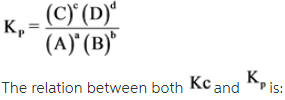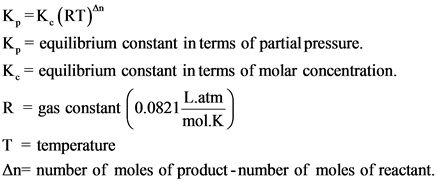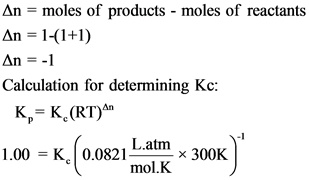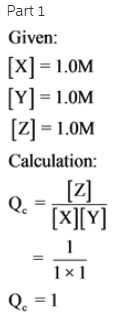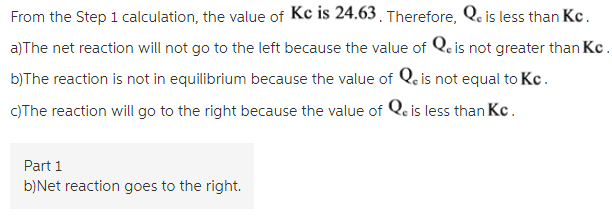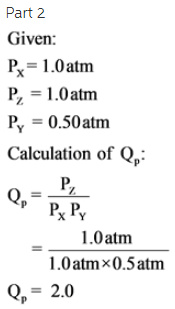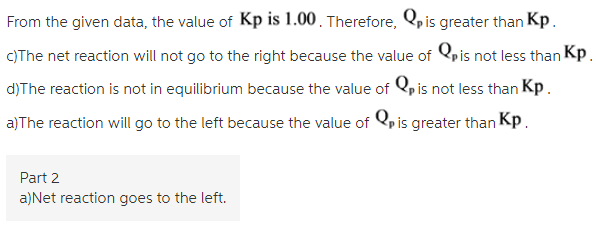# Which direction will the net reaction proceed. X(g) + Y(g) <==> Z(g) .. Kp = 1.00 at 300k

n which direction will the net reaction proceed.
X(g) + Y(g) <==> Z(g) … Kp = 1.00 at 300k
for each of these sets of initial conditions?

1) [X] = [Y] = [Z] = 1.0 M
[a] net reaction goes to the left [this one?]
[b] net reaction goes to the right
[c] reaction is at equilibrium

2. Px = Pz = 1.0 atm, Py = 0.50 atm

[a] net reaction goes to the left
[b] net reaction goes to the right
[c] reaction is at equilibrium

Concepts and reason
Equilibrium constant is the ratio between the concentration of products to the concentration of reactants. The equilibrium constant is expressed as K. If the value of K is more than 1, the equilibrium favors products and if the value of K is less than 1, the equilibrium favors reactants.

Fundamentals
For a simple equilibrium reaction,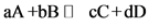The equilibrium constant with respect to molar concentration is represented as Kc.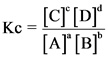The equilibrium constant in terms of partial pressure can be represented as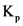.